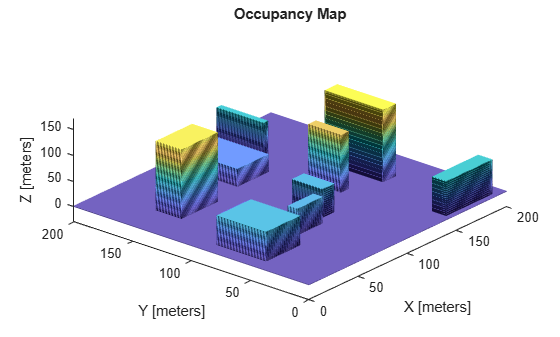# Generate Random 3-D Occupancy Map for UAV Motion Planning

This example shows how to generate a random 3-D occupancy map by automatically adding the desired number of obstacles of varying dimensions at random positions on the map. You can then use the generated map for UAV motion planning using algorithms like RRT, RRT* and Hybrid A*.

Choose a random number generator and set it's seed using the `rng` function for repeatability of the result. Change these values to obtain a different 3-D occupancy map. This step is optional. Use the seed and generator given below to reproduce the map generated in this example.

`rng(7,"twister");`

Create an empty 3-D occupancy map. Specify the maximum width and maximum length of the map as desired. In this example, the map has an area of 200-by-200 square meters.

```omap3D = occupancyMap3D; mapWidth = 200; mapLength = 200;```

Specify the number of obstacles to be added.

`numberOfObstacles = 10;`

Add the obstacles one after another using a while loop.

1. Generate the position and dimensions of the obstacle randomly using the `randi` function. Make sure the obstacle does not cross the map's boundaries.

2. Obtain the 3D grid coordinates of the obstacle using the `meshgrid` function.

3. Use the `checkOccupancy` (Navigation Toolbox) function to check if the obstacle intersects any other previously added obstacle. If it does, go to step 1. If it does not, move on to the next step.

4. Use the `setOccupancy` (Navigation Toolbox) function to set the occupancy values of the obstacle's location as 1.

```obstacleNumber = 1; while obstacleNumber <= numberOfObstacles width = randi([1 50],1); % The largest integer in the sample intervals for obtaining width, length and height length = randi([1 50],1); % can be changed as necessary to create different occupancy maps. height = randi([1 150],1); xPosition = randi([0 mapWidth-width],1); yPosition = randi([0 mapLength-length],1); [xObstacle,yObstacle,zObstacle] = meshgrid(xPosition:xPosition+width,yPosition:yPosition+length,0:height); xyzObstacles = [xObstacle(:) yObstacle(:) zObstacle(:)]; checkIntersection = false; for i = 1:size(xyzObstacles,1) if checkOccupancy(omap3D,xyzObstacles(i,:)) == 1 checkIntersection = true; break end end if checkIntersection continue end setOccupancy(omap3D,xyzObstacles,1) obstacleNumber = obstacleNumber + 1; end```

As a UAV must not collide with the ground during it's flight, consider the ground also as an obstacle. So, set the occupancy of the ground plane (x-y plane) as 1, indicating that it is an obstacle.

```[xGround,yGround,zGround] = meshgrid(0:mapWidth,0:mapLength,0); xyzGround = [xGround(:) yGround(:) zGround(:)]; setOccupancy(omap3D,xyzGround,1)```

Display the final occupancy map.

```figure("Name","3D Occupancy Map") show(omap3D)```For an example on how to use the created occupancy map, see Motion Planning with RRT for Fixed-Wing UAV.